• Physical Computing midterm project – Mirror, Mirror

Physical computing midterm project : Mirror, Mirror

Kenzo Nakamura and Tiri Kananuruk

We came up with idea of making switch for the light by clapping because we are lazy to get up and walk to switch. We decide to use Microphone Sound Detector for a sensor to catch the sound and send analog signal to the light.

first prototype used a real lamp. so we have to use relay to power up the voltage. It works! but it can’t dimming the light. The problem is the power switch tail can’t dimming the light.(because it convert DC to AC) So we have to change from light bulb to Neopixel LED

and IT BROUGHT US A LOT OF PROBLEM!!!! hahahaha oh gosh!!!!
okay first, we have to change all the code. Neopixel need their own library.

Finally our code works!! here is the final arduino code!
With helps from these people!
Thomas Igoe
Jingwen Zhu
Cristobal Valenzuela
Koji Kanao
Sebastian Morales
Richard Lapham
Rubin Huang

Thank you so much!

#include

#define PIXEL_PIN 6

#define PIXEL_COUNT 60

bool oldState = HIGH;
int showType = 0;

const int ledPinR = 11;
int soundLevel = 0; //value read from the potentiometer

int light = {0, 128, 255};
String lightPower = {“OFF”, “LOW”, “HIGH”};

int lightLevel = 0;

int colorWipe = 0;

int clapCount = 0;

long startTime;
long timeNow;
int timer = 3000;

int counter = 0;

int r = 100;
int g = 100;
int b = 100;

void setup() {
//initialize serial communications at 9600 bps:
Serial.begin(9600);

Serial.println(“hi”);
//Serial.println(sizeof(light)/sizeof(int));/// sizeof(char));
Serial.println( lightPower );
Serial.println( lightPower );
Serial.println( lightPower );
Serial.println(“yo”);

//declare the led pin as an output:
pinMode (ledPinR, OUTPUT);
strip.begin();

for (int i = 0; i < PIXEL_COUNT; i++) { strip.setPixelColor(i, strip.Color(0, 150, 0)); } strip.show(); // Initialize all pixels to 'off' } void loop() { soundLevel = analogRead(A0); //read the pot value if (soundLevel > 1000) {
if (clapCount == 0) {
startTime = millis(); // get the time of the peak
}
clapCount++;
Serial.print(clapCount);
Serial.print(“,”);
Serial.print(lightPower[lightLevel]);
Serial.print(“,”);
Serial.print(r);
Serial.print(“,”);
Serial.print(g);
Serial.print(“,”);
Serial.println(b);

delay(50);
}

timeNow = millis();

if (clapCount > 0) {
if ((timeNow – startTime) > timer) { // reset clap counter to zero
if (clapCount == 1) { // if 1 clap, shift color
if (lightLevel == 0) {

lightLevel = 2;
changeStripColor(r,g,b);

clapCount = 0;

Serial.print(clapCount);
Serial.print(“,”);
Serial.print(lightPower[lightLevel]);
Serial.print(“,”);
Serial.print(r);
Serial.print(“,”);
Serial.print(g);
Serial.print(“,”);
Serial.println(b);

Serial.print(“0”);
Serial.print(“,”);
Serial.print(lightPower[lightLevel]);
Serial.print(“,”);
Serial.print(r);
Serial.print(“,”);
Serial.print(g);
Serial.print(“,”);
Serial.println(b);
} else {
lightLevel = 0;

// analogWrite (ledPinR, light[lightLevel]);
changeStripColor(r,g,b);

clapCount = 0;

Serial.print(clapCount);
Serial.print(“,”);
Serial.print(lightPower[lightLevel]);
Serial.print(“,”);
Serial.print(r);
Serial.print(“,”);
Serial.print(g);
Serial.print(“,”);
Serial.println(b);

Serial.print(“0”);
Serial.print(“,”);
Serial.print(lightPower[lightLevel]);
Serial.print(“,”);
Serial.print(r);
Serial.print(“,”);
Serial.print(g);
Serial.print(“,”);
Serial.println(b);
}
}

if (clapCount == 2) { // if 2 claps, turn down
lightLevel–;
}
if (clapCount == 3) { // if 3 claps, turn up
lightLevel++;
}
if (lightLevel == 3) { // stay within light range
lightLevel = 2;
};
if (lightLevel < 0) { // stay within light range lightLevel = 0; }; //analogWrite (ledPinR, light[lightLevel]); //only AFTER timer is done do we measure clapIndex changeStripColor(r,g,b); clapCount = 0; Serial.print(clapCount); Serial.print(","); Serial.print(lightPower[lightLevel]); Serial.print(","); Serial.print(r); Serial.print(","); Serial.print(g); Serial.print(","); Serial.println(b); Serial.print("0"); Serial.print(","); Serial.print(lightPower[lightLevel]); Serial.print(","); Serial.print(r); Serial.print(","); Serial.print(g); Serial.print(","); Serial.println(b); } } if (clapCount == 4) { r = random (0,255); g = random (0,255); b = random (0,255); changeStripColor(r,g,b); clapCount = 0; } } void changeStripColor(int r, int g, int b) { for (int i = 0; i < PIXEL_COUNT; i++) { strip.setBrightness(light[lightLevel]); strip.setPixelColor(i, strip.Color(r, g, b)); } strip.show(); }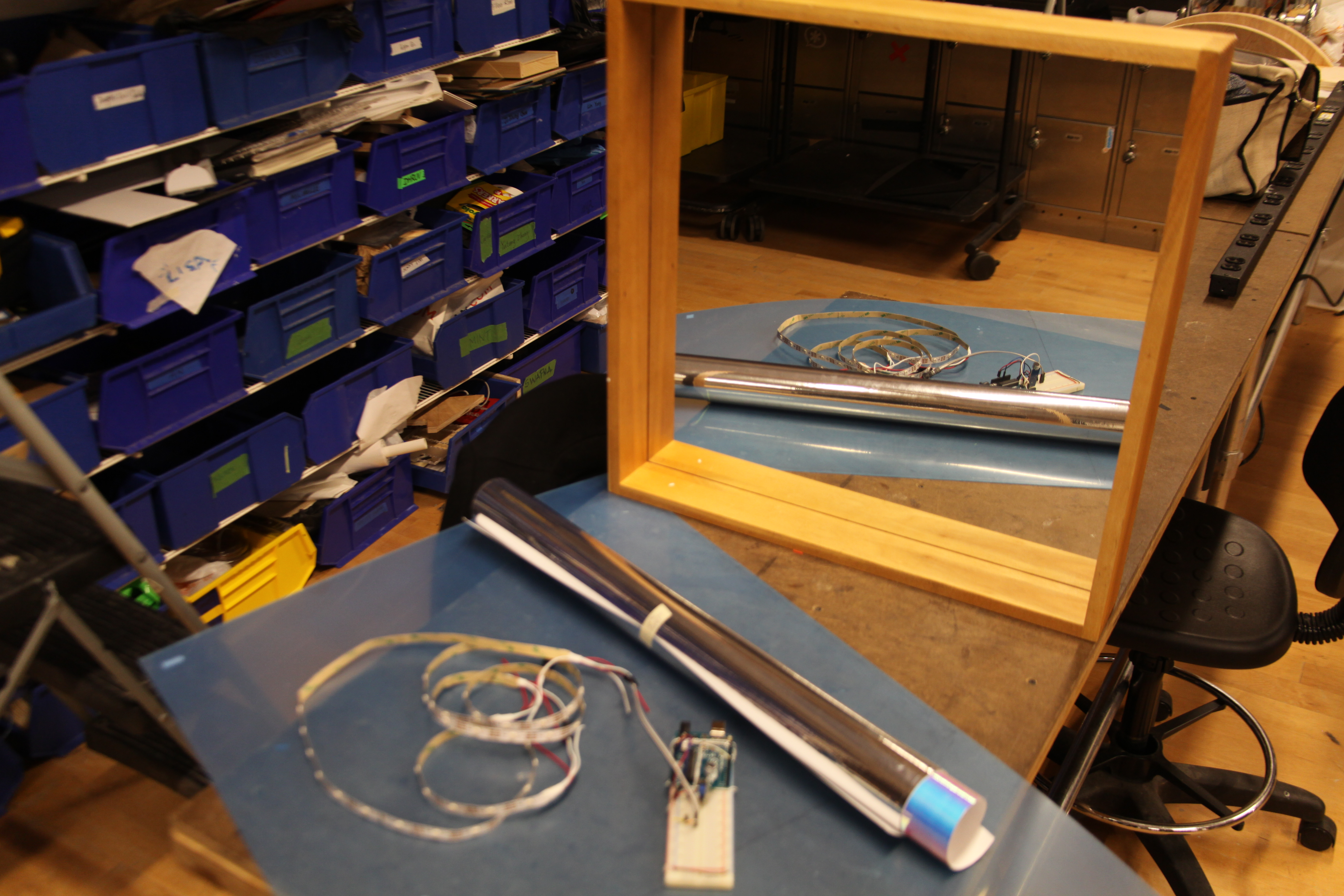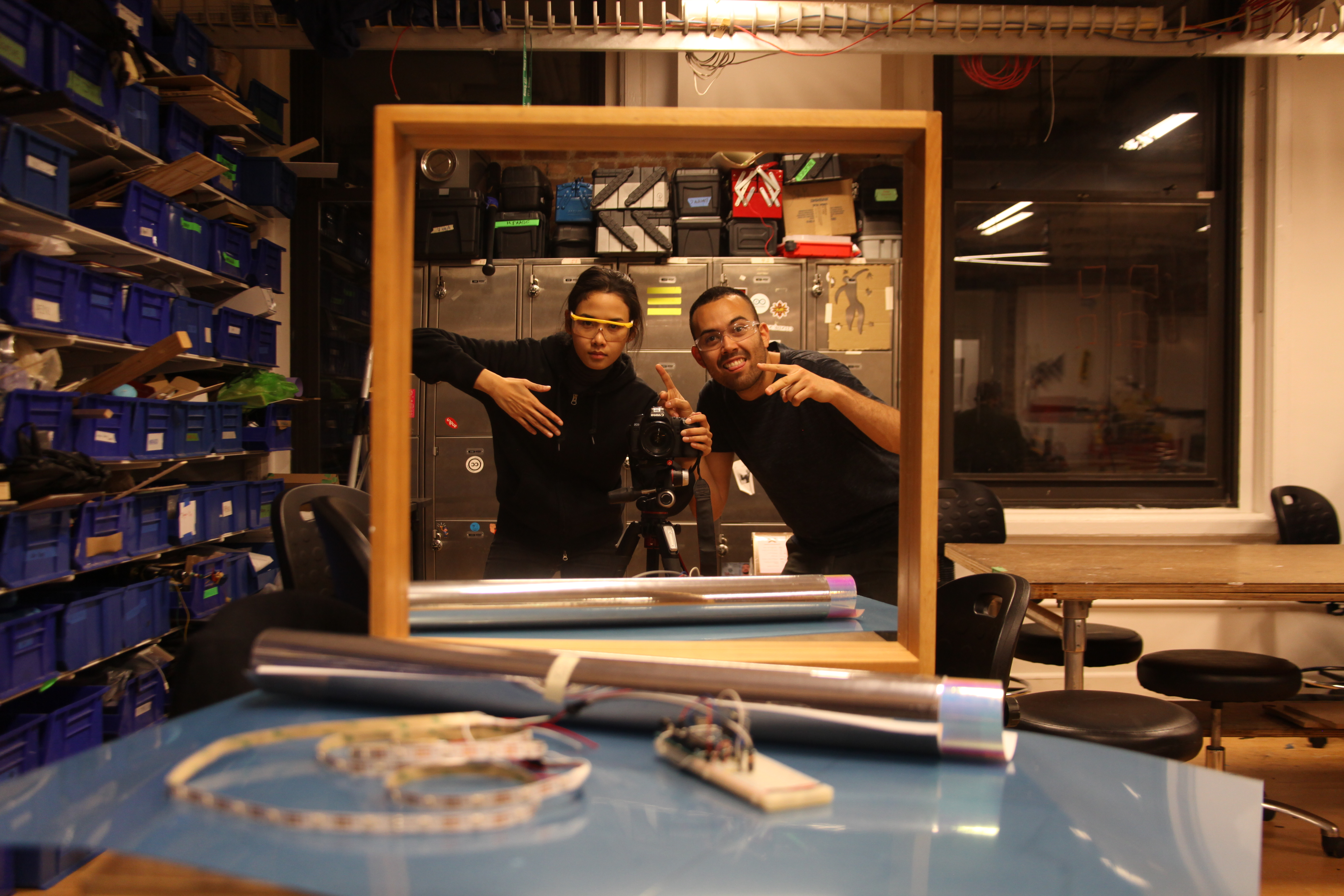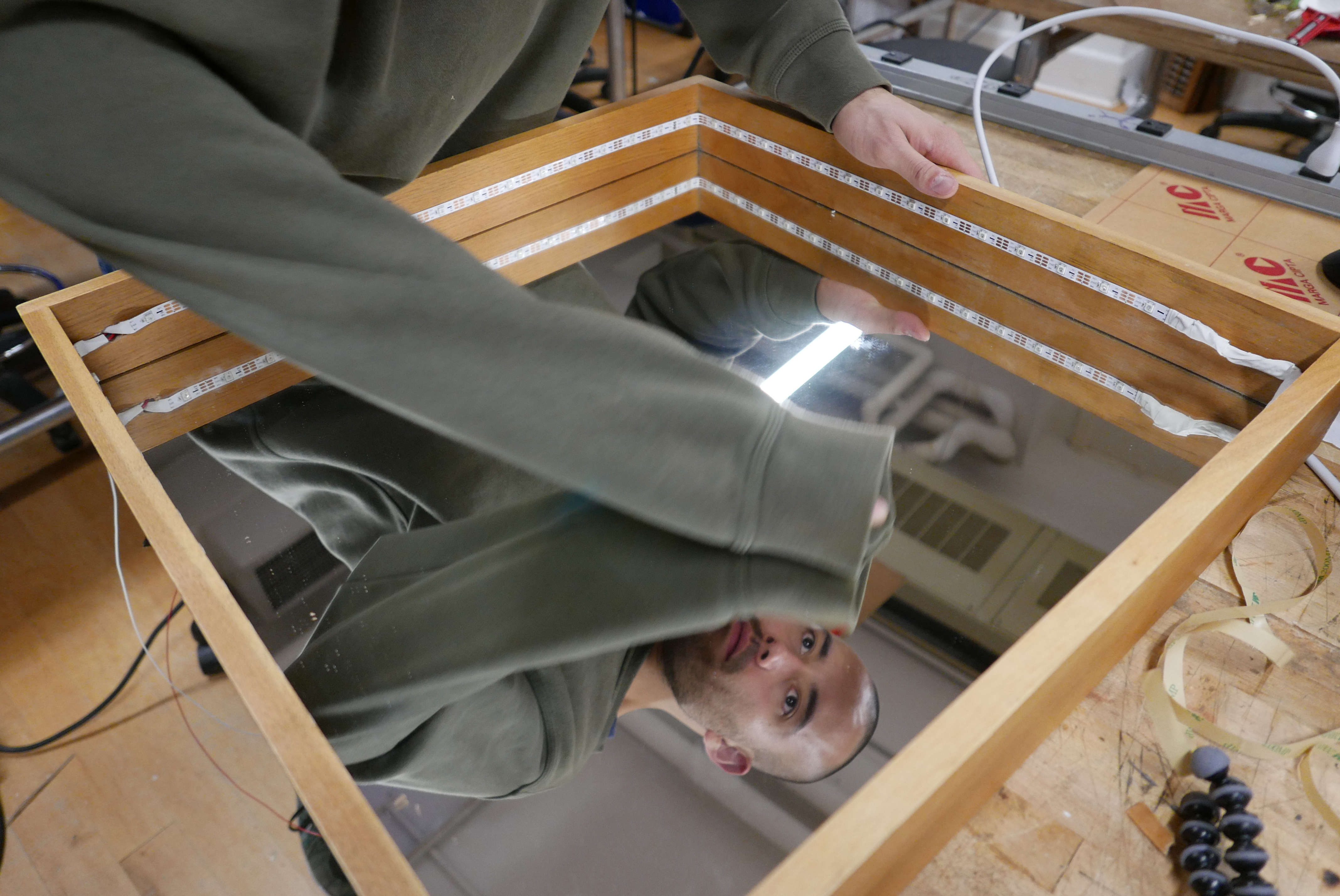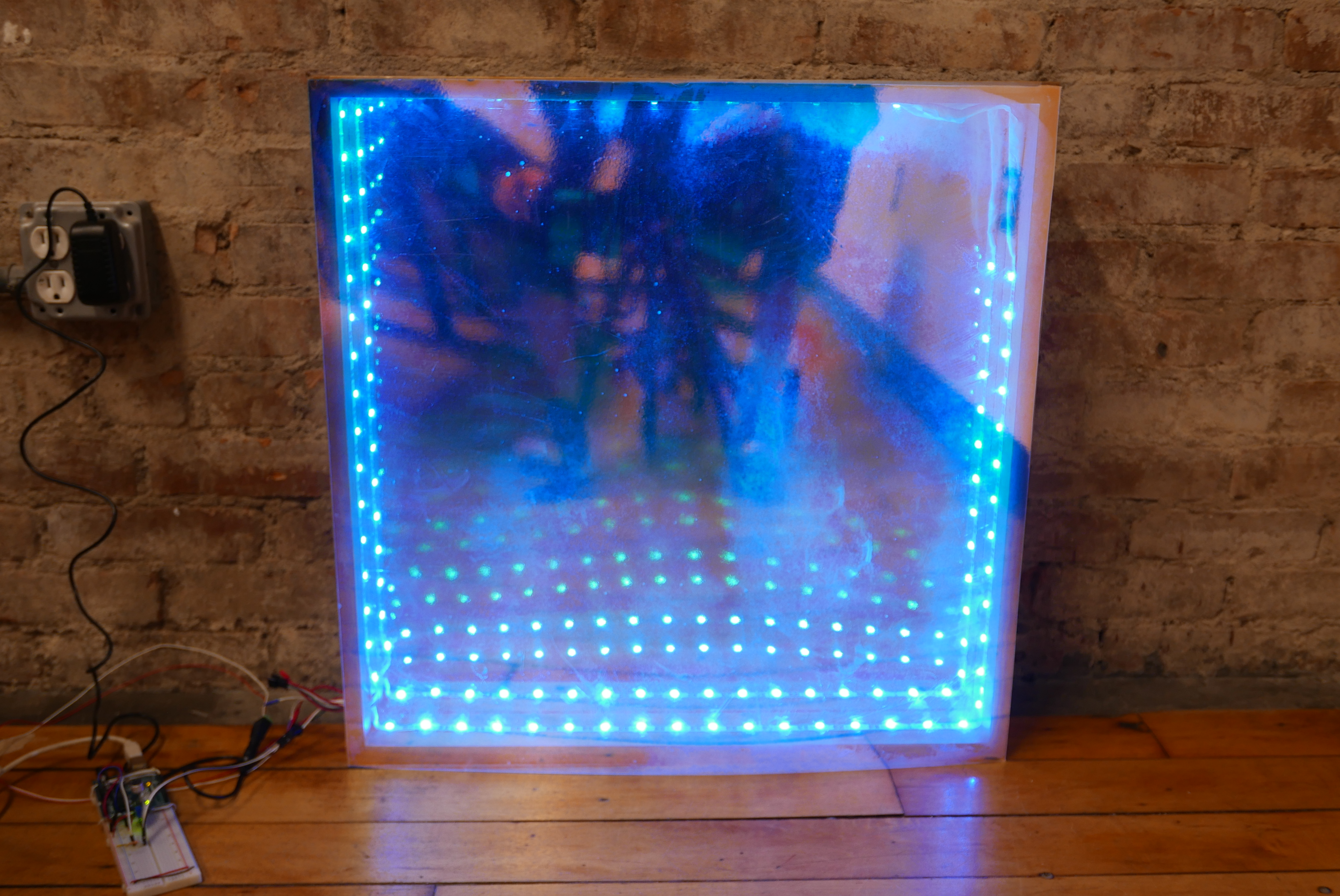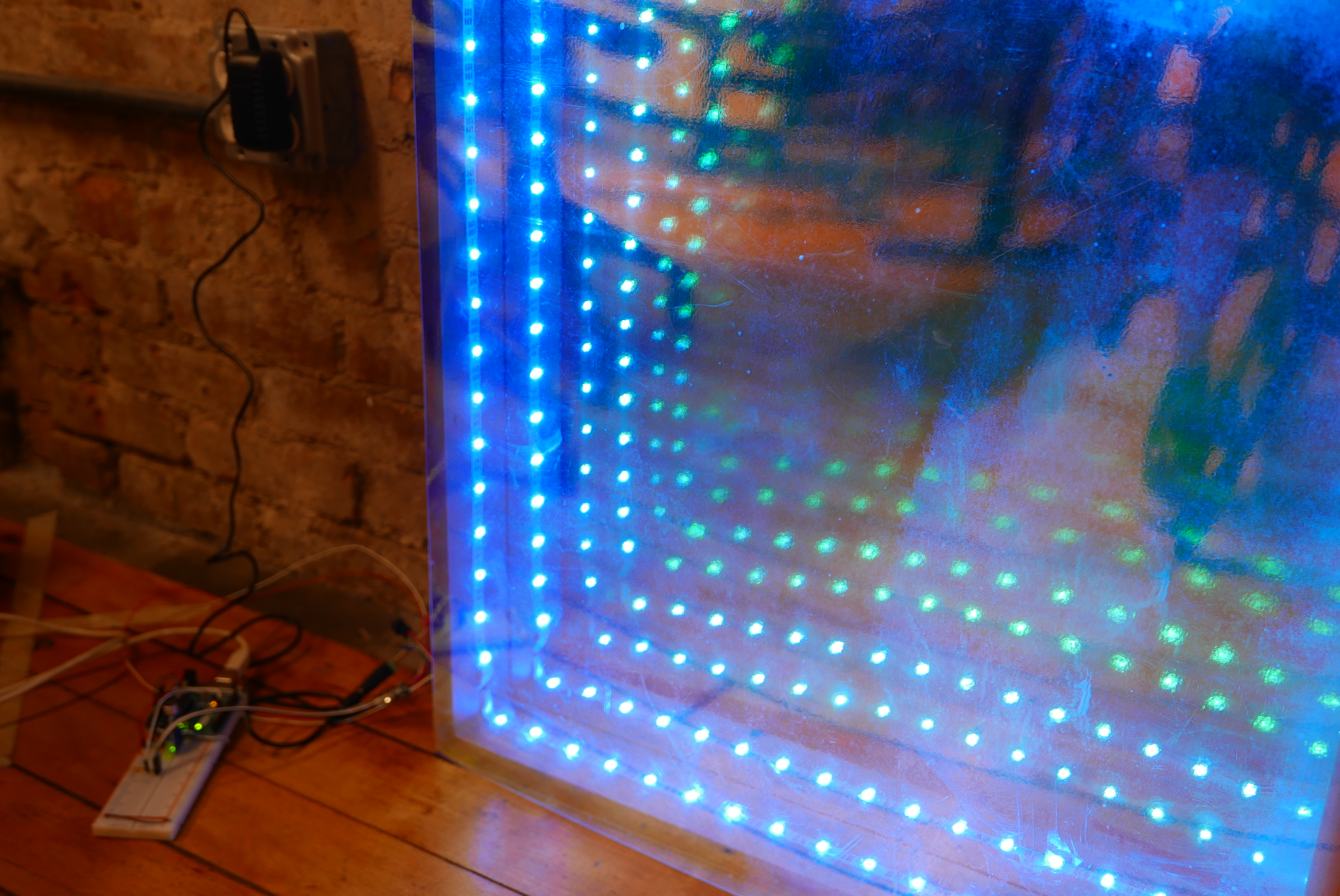Kenzo did a great job on P5 sketch! he made a Serial Input to P5! and made a cool interface

and yes Here is the final video!!

shout out to everyone who help us and give us love(and snacks!)